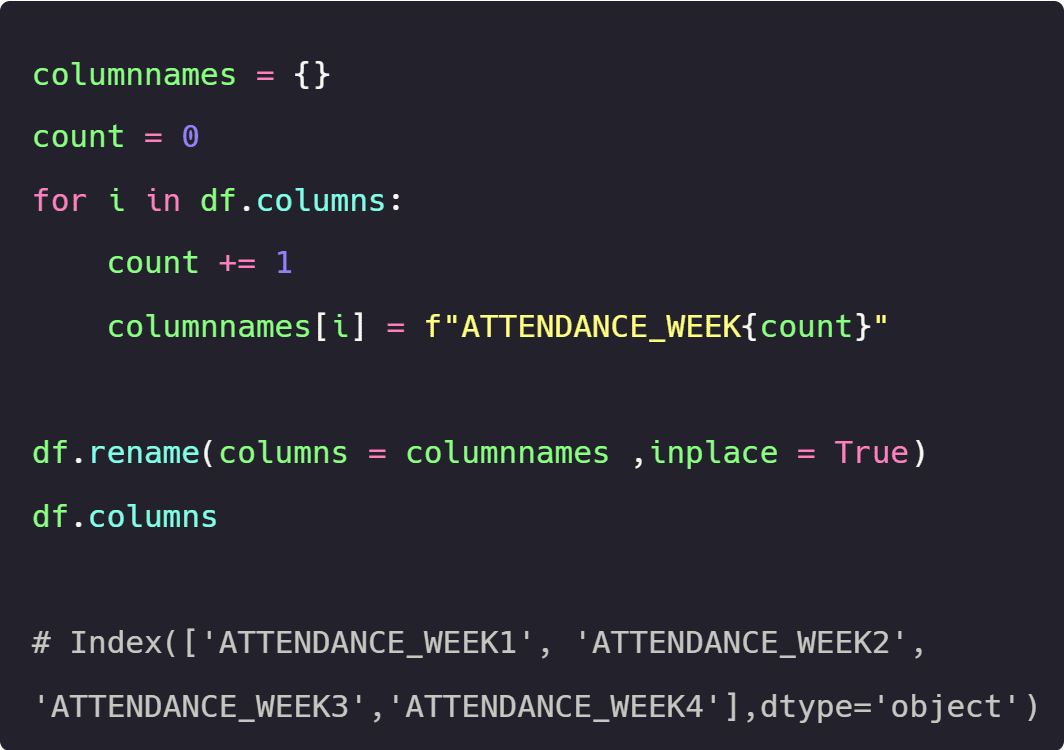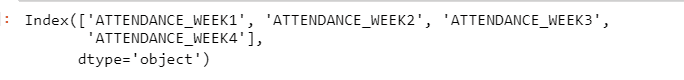# Customize Your Data Frame Column Names in Python

This tutorial will explore four scenarios in which you can apply different transformations to all DataFrame columns.Image by Editor

Be it any profession in data science apart from the regular data cleaning and model pipeline building, an individual is also required to produce results in a way which is easily interpretable to the business. In this tutorial we will explore four scenarios in which you can apply different transformations to all Data Frame columns simultaneously.

Before deep diving into the scenarios lets import pandas library and create a Data Frame named df with the following column names:

• week_one_attendance
• week_two_attendance
• week_three_attendance
• week_four_attendance

Code:

```import pandas as pd

df = pd.DataFrame (data = [[0.10,0.20,0.70,0.80],[0.80,0.50,0.40,0.20],[0.50,0.10,0.20,0.10],[0.30,0.45,0.97,0.65]],

columns = ["week_one_attendance","week_two_attendance","week_three_attendance","week_four_attendance"])

df```

Output:LET’S GET KICKSTARTED WITH THE SCENARIOS

# Scenario 1

In the code below a for loop is used to iterate over all the columns of the Data Frame, where in every iteration each column is converted to upper case using the rename method

Code:

```for i in df.columns:

df.rename(columns = {i:i.upper()},inplace = True)

df.columns```

Output:# Scenario2

In the code below we will be declaring an empty dictionary named columnnames and then declare another variable named count to 0

We would then be using for loop to iterate over all the columns of the Data Frame, where in every iteration the count variable would be incremented by 1. The incremented value would then be used inside the fstring to generate a new column name. The original and new column names will be added to the dictionary as key, value pairs in every iteration.

After constructing the dictionary columnnames with the original and new column names we will then passing the dictionary to the rename  method

Code:

```columnnames = {}

count = 0

for i in df.columns:

count += 1

columnnames[i] = f"WEEK_{count}_ATTENDANCE"

columnnames```

Output:Code:

```df.rename(columns = columnnames ,inplace = True)

df.columns```

Output:# Scenario 3

In the code below we will declare an empty dictionary named columnnames

We would then be using for loop  to iterate over all the columns of the Data Frame, where in every iteration the first occurrence of the underscore will be replaced by no space. The original and new column names will be added to the dictionary as key, value pairs in every iteration.

After constructing the dictionary columnnames with the original and new column names, we will then passing the dictionary to the rename  method

Code:

```columnnames = {}

for i in df.columns:

x = i.replace('_','',1)

columnnames[i] = x

columnnames```

Output:Code:

```df.rename(columns = columnnames ,inplace = True)

df.columns```

Output:# Scenario 4

In the code below we will be declaring an empty dictionary named columnnames and then declare another variable named count to 0

We would then be using for loop to iterate over all the columns of the Data Frame, where in every iteration the count variable would be incremented by 1. The incremented value would then be used inside the fstring to generate a new column name having the positions of the first and last word swapped. The original and new column names will be added to the dictionary as key, value pairs in every iteration.

After constructing the dictionary column names with the original and new column names we will then passing the dictionary to the rename  method

Code:

```columnnames = {}

count = 0

for i in df.columns:

count += 1

columnnames[i] = f"ATTENDANCE_WEEK{count}"

columnnames```

Output:Code:

```df.rename(columns = columnnames ,inplace = True)

df.columns```

Output:# Conclusion

Rather than manually updating every column name one by one, by using for loops and different methods available with Python Strings we were able to update the values of all the columns of a Data Frame simultaneously hence saving an ample amount of time

Priya Sengar (Medium, Github) is a Data Scientist with Old Dominion University. Priya is passionate about solving problems in data and converting them into solutions.

By subscribing you accept KDnuggets Privacy Policy

By subscribing you accept KDnuggets Privacy Policy

By subscribing you accept KDnuggets Privacy Policy